Next: Inverse of the overlap Up: Theory Previous: Theory

## Isospin projection formalism

Within the MF approximation, the isospin symmetry breaking has two sources. The spontaneous breaking of isospin associated with the MF approximation itself [36,26,29] is, in this theory, intertwined with the explicit symmetry breaking due to the Coulomb interaction. The essence of our method [28,29] is to retain the explicit isospin mixing. It is achieved by rediagonalizing the total effective nuclear Hamiltonian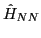in good-isospin basis. The basis is generated by using the projection-after-variation technique, that is, by acting with the standard one-dimensional isospin-projection operator on the MF product state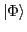: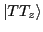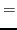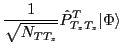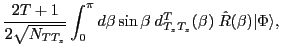(1)

where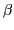denotes the Euler angle associated with the rotation operator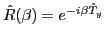about the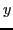-axis in the isospace,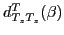is the Wigner function , and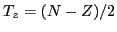is the third component of the total isospin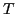. The wave functionis obtained self-consistently by solving the Skyrme-Hartree-Fock (SHF) equations. In the HF limit, the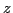-component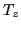is strictly conserved. The overlap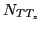, or interchangeably the normalization factor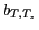, are given by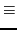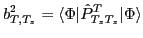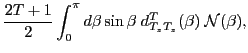(2)

where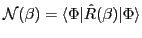(3)

stands for the overlap kernel. The good-isospin basis is spanned by the states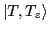that have tangible contributions to the MF state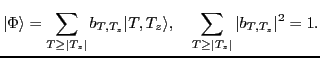(4)

In practice, we retain states having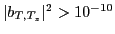, which sets the limit of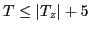.

The total nuclear Hamiltonian consists of the kinetic energy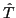, the Skyrme interaction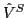and the Coulomb interaction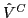that breaks isospin: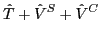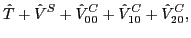(5)

where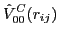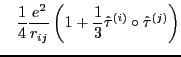(6)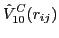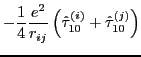(7)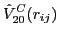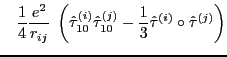(8)

are, respectively, isoscalar, covariant rank-1 (isovector), and covariant rank-2 axial (isotensor) spherical tensor components of the Coulomb interaction. They are constructed by coupling spherical components of the one-body isospin operator: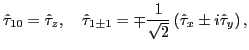(9)

where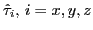denote Pauli matrices and symbol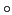stands for the scalar product of isovectors.

The Coulomb interaction is the only source of the isospin symmetry violation in our model. Charge symmetry breaking components of the strong interaction and the isovector kinetic energy (which is quenched as compared to its isoscalar counterpart by a factor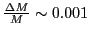due to very small mass difference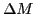between the neutron and the proton relative to mean nucleonic mass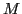) are not taken into account. Hence, the isoscalar part of the total Hamiltonian reads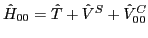. Its matrix elements can be cast into one-dimensional integrals: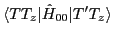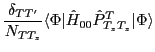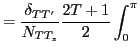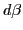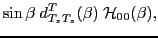(10)

involving the Hamiltonian kernel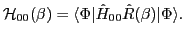(11)

Calculation of matrix elements of the isovector (7) and isotensor (8) components of the Coulomb interaction is slightly more complicated. It appears, however, that these matrix elements can also be reduced to one-dimensional integrals over the Euler angle: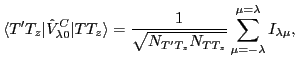(12)

where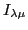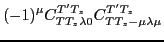(13)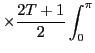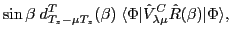and symbols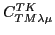stand for the Clebsch-Gordan coefficients . The non-axial (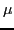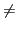0) isovector and isotensor components of the Coulomb interaction appearing in Eq. (13) are: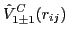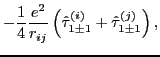(14)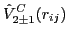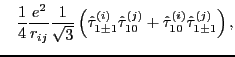(15)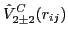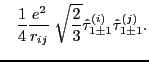(16)

Equations (12)-(13) can be derived by using the standard transformation rules for a covariant spherical-tensor operator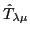of rank-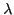under the three-dimensional rotation by the Euler angles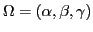, that is,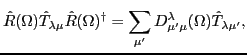(17)

where the SO(3) projection operator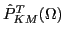commutes with spherical tensors as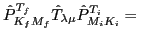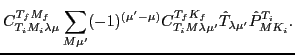(18)

The cornerstone of the isospin projection scheme described above is a calculation of the Hamiltonian and norm kernels and subsequent one-dimensional integration over the Euler angle. The integrals are calculated numerically using the Gauss-Legandre quadrature, which is very well suited for this problem provided that the calculated kernels are non-singular.Next: Inverse of the overlap Up: Theory Previous: Theory
Jacek Dobaczewski 2010-01-30